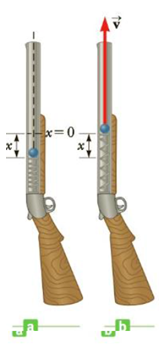Chapter 5, Problem 39P

Chapter
Section
Textbook Problem

The launching mechanism of a toy gun consists of a spring of unknown spring constant, as shown in Figure P5.39a. If the spring is compressed a distance of 0.120 m and the gun fired vertically as shown, the gun can launch a 20.0-g projectile from rest to a maximum height of 20.0 m above the starting point of the projectile. Neglecting all resistive forces, (a) describe the mechanical energy transformations that occur from the time the gun is fired until the projectile reaches its maximum height, (b) determine the spring constant, and (c) find the speed of the projectile as it moves through the equilibrium position of the spring (where x = 0), as shown in Figure P5.39b.Figure P5.39

(a)

To determine
The mechanical energy transformation.

Explanation

Before the gun is fired all the energy is stored in the form of elastic potential energy in the spring. Just after the gun is fired, when the bullet starts its projectile motion; most of the energy that was stored as elastic potential in the spring will change to the kinetic energy of the bullet.

Some part of the energy wil converts to gravitational potential energy. When the bullet goes up the speed of the bullet and there by kinetic energy of the bullet will be decreasing accordingly. However, when the bullet goes up, as the height increases; the gravitational potential energy of the bullet will be increasing...

(b)

To determine
The spring constant.

(c)

To determine
The speed of the projectile as it moves through the equilibrium position of the spring.

Still sussing out bartleby?

Check out a sample textbook solution.

See a sample solution

The Solution to Your Study Problems

Bartleby provides explanations to thousands of textbook problems written by our experts, many with advanced degrees!

Get Started